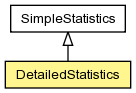## org.jboss.dna.common.statistic Class DetailedStatistics<T extends Number>

```java.lang.Objectorg.jboss.dna.common.statistic.SimpleStatistics<T>org.jboss.dna.common.statistic.DetailedStatistics<T>
```
Type Parameters:
`T` - the number type for these statistics

```@ThreadSafe
public class DetailedStatistics<T extends Number>extends SimpleStatistics<T>```Encapsulation of the statistics for a series of values to which new values are frequently added. The statistics include the `minimum`, `maximum`, `total (aggregate sum)`, `mean (average)`, `median`, `standard deviation` and the `histogram` of the values.

This class uses an efficient running calculation of the mean and standard deviation that is not as susceptible to roundoff errors as other traditional algorithms. The recursive algorithm is as follows, where M is the median value, sigma is the standard deviation, and S is a variable used in the calculation of sigma:

```   M(1) = x(1)
S(1) = 0
M(k) = M(k-1) + ( x(k) - M(k-1) ) / k
S(k) = S(k-1) + ( x(k) - M(k-1) ) * (x(k) - M(k))
```
Then, the standard deviation for n values in x is
``` sigma = sqrt(S(n) / n)
```

Unlike the other quantities, the median value (the value at which half of the values are greater and half the values are lower) cannot be calculated incrementally. Therefore, this class does record the values so that the median can be properly calculated. This fact should be kept in mind when performing statistics on large numbers of values.

Constructor Summary
`DetailedStatistics(MathOperations<T> operations)`

Method Summary
` Histogram<T>` `getHistogram()`
Return the histogram of the `values`.
` Histogram<T>` `getHistogram(int numSigmas)`
Return the histogram of the `values`.
` T` `getMedian()`
Return the approximate mean (average) value represented as an instance of the operand type.
` double` `getMedianValue()`
Return the median value.
` double` `getStandardDeviation()`
Return the standard deviation.
` List<T>` `getValues()`
Get the values that have been recorded in these statistics.
` String` `toString()`

Methods inherited from class org.jboss.dna.common.statistic.SimpleStatistics
`add, getCount, getMathOperations, getMaximum, getMean, getMeanValue, getMinimum, getTotal, reset`

Methods inherited from class java.lang.Object
`equals, getClass, hashCode, notify, notifyAll, wait, wait, wait`

Constructor Detail

### DetailedStatistics

`public DetailedStatistics(MathOperations<T> operations)`
Method Detail

### getValues

`public List<T> getValues()`
Get the values that have been recorded in these statistics. The contents of this list may change if new values are `added` in another thread.

Returns:
the unmodifiable collection of values, in insertion order

### getMedian

`public T getMedian()`
Return the approximate mean (average) value represented as an instance of the operand type. Note that this may truncate if the operand type is not able to have the required precision. For the accurate mean, see `getMedianValue()`.

Returns:
the mean (average), or 0.0 if the `count` is 0

### getMedianValue

`public double getMedianValue()`
Return the median value.

Returns:
the median value, or 0.0 if the `count` is 0
`getMedian()`

### getStandardDeviation

`public double getStandardDeviation()`
Return the standard deviation. The standard deviation is a measure of the variation in a series of values. Values with a lower standard deviation has less variance in the values than a series of values with a higher standard deviation.

Returns:
the standard deviation, or 0.0 if the `count` is 0 or if all of the values are the same.

### getHistogram

`public Histogram<T> getHistogram()`
Return the histogram of the `values`. This method returns a histogram where all of the buckets are distributed normally and all have the same width. In this case, the 'numSigmas' should be set to 0. For other variations, see `getHistogram(int)`.

Returns:
the histogram
`getHistogram(int)`

### getHistogram

`public Histogram<T> getHistogram(int numSigmas)`
Return the histogram of the `values`. This method is capable of creating two kinds of histograms. The first kind is a histogram where all of the buckets are distributed normally and all have the same width. In this case, the 'numSigmas' should be set to 0. See `getHistogram()`.

The second kind of histogram is more useful when most of the data that is clustered near one value. This histogram is focused around the values that are up to 'numSigmas' above and below the `median`, and all values outside of this range are placed in the first and last bucket.

Parameters:
`numSigmas` - the number of standard deviations from the `median`, or 0 if the buckets of the histogram should be evenly distributed
Returns:
the histogram
`getHistogram()`
`public String toString()`
`toString` in class `SimpleStatistics<T extends Number>`